Processing ......FreeComputerBooks.com Links to Free Computer, Mathematics, Technical Books all over the World

Fundamental Numerical Methods and Data Analysis
Top Free Unix/Linux Books 🌠 - 100% Free or Open Source!
• Title Fundamental Numerical Methods and Data Analysis
• Author(s) George W. Collins, II
• Publisher: Harvard University Press (2003)
• Paperback N/A
• eBook PDF
• Language: English
• ISBN-10: N/A
• ISBN-13: N/A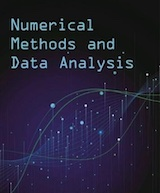Book Description

The basic premise of this book is that it can serve as the basis for a wide range of courses that discuss numerical methods used in data analysis and science. It is meant to support a series of lectures, not replace them. To reflect this, the subject matter is wide ranging and perhaps too broad for a single course.

Numerical algorithms appear as neatly packaged computer programs that are regarded by the user as "black boxes" into which they feed their data and from which come the publishable results. The complexity of many of the problems dealt with in this manner makes determining the validity of the results nearly impossible. This book is an attempt to correct some of these problems.

• N/A
Reviews, Ratings, and Recommendations: Related Book Categories: Read and Download Links:Similar Books:
•Tea Time Numerical Analysis: Experiences in Mathematics

This textbook was born of a desire to contribute a viable, free, introductory Numerical Analysis textbook. The ultimate goal of this book is to be a complete, one-semester, single-pdf, downloadable textbook designed for mathematics classes.

•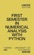First Semester in Numerical Analysis with Python (Yaning Liu)

This book introduces students to Numerical Methods using Python for the implementation of the algorithms. Discusses several common applications of Numerical Analysis and implementation using real world examples and hands on programming exercises.

•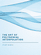The Art of Polynomial Interpolation (Stuart Murphy)

Exploring the techniques of interpolating data allows us to view the development and birth of a polynomial. This book is focused on laying a foundation for understanding and applying several common forms of Polynomial Interpolation.

•Numerical Algorithms: Computer Vision, Machine Learning, etc.

This book presents a new approach to numerical analysis for modern computer scientists, covers a wide range of topics - from numerical linear algebra to optimization and differential equations - focusing on real-world motivation and unifying themes.

•Numerical Methods and MATLAB Programming for Engineers

It covers the fundamentals while emphasizing the most essential numerical methods. Readers will enhance their programming skills using MATLAB to implement algorithms, discover how to use MATLAB to solve problems in science and engineering.

•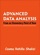Advanced Data Analysis from an Elementary Point of View

This is a textbook on data analysis methods, intended for advance undergraduate students who have already taken classes in probability, mathematical statistics, and linear regression. It presumes that you can read and write simple functions in R.

•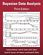Bayesian Data Analysis (Andrew Gelman, et al.)

This classic book is widely considered the leading text on Bayesian methods, lauded for its accessible, practical approach to analyzing data and solving research problems. It takes an applied approach to analysis using up-to-date Bayesian methods.

•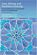Data Mining and Analysis: Fundamental Concepts and Algorithms

This textbook provides a broad yet in-depth overview of data mining, integrating related concepts from machine learning and statistics. The main parts of the book include exploratory data analysis, pattern mining, clustering, and classification.

•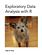Exploratory Data Analysis with R (Roger D. Peng)

This book covers the essential exploratory techniques for summarizing data with R. These techniques are typically applied before formal modeling commences and can help inform the development of more complex statistical models.

•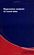Basic Data Analysis and More - A Guided Tour using Python

In this book, a selection of frequently required statistical tools will be introduced and illustrated. An exemplary implementation of the presented techniques using the Python programming language is provided.

Book Categories
 :All CategoriesTop Free BooksRecent BooksMiscellaneous BooksComputer EngineeringComputer LanguagesComputer ScienceData Science/DatabasesJava and Java EE (J2EE)Linux and UnixMathematicsMicrosoft and .NETMobile ComputingNetworking and CommunicationsSoftware EngineeringSpecial TopicsWeb Programming
Other Categories